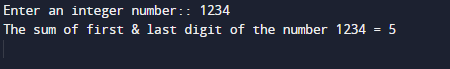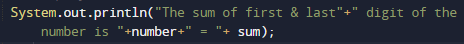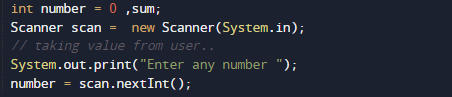# Java Program to Find Sum of first & last digit of a number

In this tutorial you will learn about the Java Program to Find Sum of first & last digit of a number and its application with practical example.

In this tutorial, we will learn to create a Java Program to Find Sum of first & last digit of a number  using Java programming.

## Prerequisites

Before starting with this tutorial we assume that you are best aware of the following Java programming topics:

• Java Operators.
• Basic Input and Output function in Java.
• Class and Object in Java.
• Basic Java programming.
• If-else statements in Java.
• For loop in Java.

## Java Program to Find Sum of first & last digit of a number.

In this program we will find sum of first and last Digit of a Number .We would first declared and initialized the required variables. Next, we would prompt user to input a digit. Later we will find sum of first and last value of a digit.

## OutputIn our program we take a digit from user and add first and last digit of a number and return the sum of first and last digit.In the above program, we have first declared and initialized a set variables required in the program.

• number=to take value from user.
• first=it will hold digits first number.
• last= it will hold digit last number.
• sum = for counting sum of first and last.
• count = for counting digit number.In the above program, we have first declared and initialized a set variables required in the program. after that we will take a value from user and find sum of its first and last digits.

How to find the sum of first and last digit of a number in java,

1. First of all take a number from user.
2. Declaring variable and initialize them.
3. First find the last digit simply we use %(modulus) operator.
4. The expression is “number %10” gives the last digit of the number.
5. For finding the first digit of a number.
6. Find the total number of digits in the given number.
1. Divide the number by `10^(total_number_of_digits-1)`, it will give the first digit of the number.Add first and the last digit to the sum variable

In this tutorial we have learn about the Java Program to Find Sum of first & last digit of a number and its application with practical example. I hope you will like this tutorial.